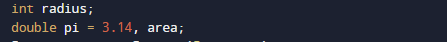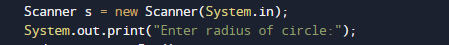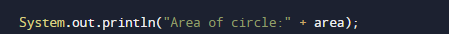# Java Program to Calculate the Area of a Circle

In this tutorial you will learn about the Java Program to Calculate the Area of a Circle and its application with practical example.

In this tutorial, we will learn to create a Java program that will Calculate Area of Cricle using Java programming.

## Prerequisites

Before starting with this tutorial we assume that you are best aware of the following Java programming topics:

• Java Operators.
• Basic Input and Output function in Java.
• Class and Object in Java.
• Basic Java programming.

## What is Area of Circle?

In Mathematics, the space occupied by the circle in a two-dimensional plane enclosed by a circle of radius r so area of circle is = (π r*r). where  π  is a Greek word that represents the constant ratio of the circumference of any circle which is  approx equal to 3.1416.

## Java Program to Calculate the Area of a Circle

In this program , we will calculate Area of Circle of a given Radius .First of all user will be prompted to enter Radius and afterword passing these value to formula to calculate Area of Circle.

## OutputIn the above program, we have first declared and initialized a set variables required in the program.

• radius = it will hold entered length.
• area=  it will hold the result i.e Area or Circle.

Area of a Circle is a product  π (which is constant) and Multiplication of radius twice r*r  So,to calculate the area of a Circle.First we will Declare variables as shown in below image.After that take value of radius from user .And using known formula of area of circle which is (π r*r) where π is a constant and r is radiushere we use “pi” in place or symbol of “π” which is constant and calculate the area of  circle of  given radius by user.At the end we will calculate area of Circle and print the value area.In this tutorial we have learn about the Java Program to Calculate the Area of a Circle and its application with practical example. I hope you will like this tutorial.